# Hankel matrix

(diff) ← Older revision | Latest revision (diff) | Newer revision → (diff)

A matrix whose entries along a parallel to the main anti-diagonal are equal, for each parallel. Equivalently,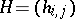is a Hankel matrix if and only if there exists a sequence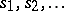, such that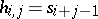,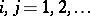. Ifare square matrices, thenis referred to as a block Hankel matrix. Infinite Hankel matrices are associated with the representation of Hankel operators acting on the Hilbert space of square summable complex sequences.

Hankel matrices are frequently encountered in applications where the close interplay between polynomial and matrix computations is exploited in order to devise very effective numerical solution algorithms. Famous special cases are the Hilbert matrix and Hilbert–Hankel operator, defined by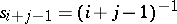, which play an important role in the study of spectral properties of integral operators of Carleman type (cf. also Carleman operator). For theoretical properties of Hankel matrices, see [a13]. For an overview of Hankel operators, see [a20]. For references to the varied and numerous relations among Hankel matrices and polynomial computations, see [a24], [a9], [a4].

To a Hankel operator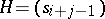one naturally associates the function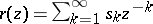, which is called its symbol. Kronecker's theorem [a9] says thathas finite rankif and only if its symbol is a rational function, that is,, whereandare mutually prime polynomials. In this caseis the number of poles of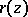and, therefore, the degree of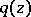. In addition, assuming thatis theleading principal submatrix of, i.e., the submatrix made up by the entries in the firstrows and columns of, thenis non-singular, whereasis singular for any.

Given a Hankel operatorwith symbol, then the problem of finding two polynomials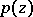andof degree at mostand, respectively, such that(a1)

is a particular instance of the Padé approximation problem. If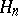is non-singular, these polynomials are uniquely determined up to a suitable normalization, say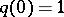, and their computation essentially amounts to solving a linear system with coefficient matrix. See [a1], [a3] and [a10] for a survey of both the theory and applications of general Padé approximation problems. In [a19] this theory is first generalized and then applied to the inversion of (block) Hankel matrices.

Other variants of (a1) can also be considered, generally leading to different computational problems. From a system-theoretic point of view, the possibility of recovering a rational function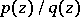, whereis monic, by its MacLaurin expansion at infinity has been extensively studied as the partial realization problem of system theory (see, for instance, [a11]). It is intimately connected to such topics as the Berlekamp–Massey algorithm in the context of coding theory and Kalman filtering. For applications of the theory of Hankel matrices to engineering problems of system and control theory, see [a14] and [a7].

The connection between Hankel matrices and orthogonal polynomials arises in the solution of moment problems (cf. also Moment problem). Given a positive Borel measureon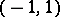, then the Hankel operatordefined by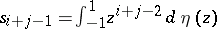,, is positive definite and, moreover, the last columns of,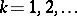, generate a sequence of orthogonal polynomials linked by a three-term recurrence relation. The converse is known as the Hamburger moment problem (cf. also Moment problem). The underlying theory is very rich and can be suitably extended to both finite Hankel matrices, by considering discrete measures, and to indefinite Hankel matrices, by means of formal orthogonal polynomials. A survey of results on Hankel matrices generated by positive measures can be found in [a22]. See [a8] and [a12] for an introduction to the theory of formal orthogonal polynomials in the context of the algorithms of numerical analysis, including Lanczos' tridiagonalization process, rational interpolation schemes, the Euclidean algorithm, and inverse spectral methods for Jacobi matrices.

Since orthogonal polynomials on the real axis generate Sturm sequences (cf. also Sturm theorem), it follows that the use of quadratic forms associated with Hankel matrices provides a means for solving real root counting problems and real root localization problems; see [a18] and [a2]. Moreover, certain properties of sequences of Hankel determinants give the theoretical bases on which both Koenig's method and the Rutishauser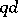algorithm, for the approximation of zeros and poles of meromorphic functions, rely; see [a26].

The problem of inverting a finite non-singular Hankel matrixhas been extensively studied in the literature on numerical methods and the connections shown earlier between the theory of Hankel matrices and many other fields have been exploited in order to derive many different Hankel system solvers.

As mentioned above (the Kronecker's theorem), if the Hankel operatorhas a rational symbolwithandmutually prime andof degree, thenis invertible. On the other hand, ifis an invertible finite Hankel matrix of orderdetermined by its entries,, then the rational function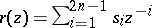can uniquely be extended to a power series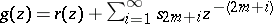in such a way that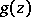is the expansion at infinity of, whereandare mutually prime withmonic of degree. In this case the inverse ofis given by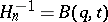, where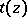is a polynomial of degree less thanthat satisfies the Bezout equation, and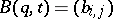denotes the Bezout matrix, whose entries are defined by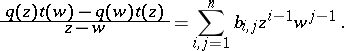Since the Bezout equation can be solved by means of the Euclidean algorithm, this brings up the possibility of a recursive approach to the inversion of Hankel matrices. Analogously, in a matrix setting this recursive structure is fully exploited by the observation that Hankel matrices have low displacement rank in a sense first defined in [a15]. Based on these properties, many fast algorithms for solving Hankel linear systems with cost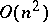have been developed. Superfast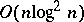algorithms have also appeared; see [a25], [a16], [a4], [a24], [a5] and [a17] for extensive bibliographies on these methods. Generalizations to the case wherehas entries over an integral domain are discussed in [a23], where the subresultant theory [a6] is described in terms of factorization properties of Hankel matrices. This becomes significant for the efficient solution of many problems in real algebraic geometry involving polynomials and rational functions [a21].

How to Cite This Entry:
Hankel matrix. Encyclopedia of Mathematics. URL: http://encyclopediaofmath.org/index.php?title=Hankel_matrix&oldid=18576
This article was adapted from an original article by Luca Gemignani (originator), which appeared in Encyclopedia of Mathematics - ISBN 1402006098. See original article